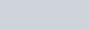• 46
•
• 2
•
• 2
•
•
•
50
Shares

# WORK AND WAGES PROBLEMS TRICKS IN HINDI1). Total wages = total days × wage of one day

2). If X, Y and Z can do work in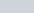and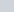days, then the ratios of their wages is =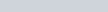3). If A and B can do a piece of work in x and y days, and their salaries are in the ratio y : x, then the wages of A and B are respectively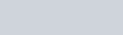4). If A, B, and C can do a piece of work in x, y, and z days, and their salaries are in the ratio yz : xz: xy, then the wages of A, B, and C are respectively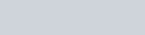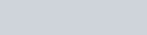5). Total salary earned by a certain person by doing a certain job = (wage of 1 person of 1 day) × (numbers of persons) × (numbers of days)
then the number of persons required =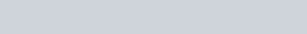6). A can do a piece of work in x days, and with the help of B, A can do a piece of work in y days, then A’s share and B’s share, if they get ₹ a.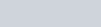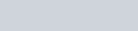7). If X, Y, Z carry a fleet to do a given work for ₹ R if both X and Y together can do only m/n part of the whole work, and Z alone does the rest, then the share of Z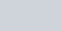# PARTNERSHIP PROBLEMS TRICKS IN HINDI1). Ratio of the amount invested by partnership = Ratio of profit share

2). If some persons do business in partnership by investing different sums of money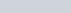at different times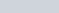, then the ratio of their profits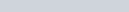3). If the ratio of profits of the partnership is x : y : z, then the ratio of theirs time in investment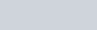And ratio of theirs sums